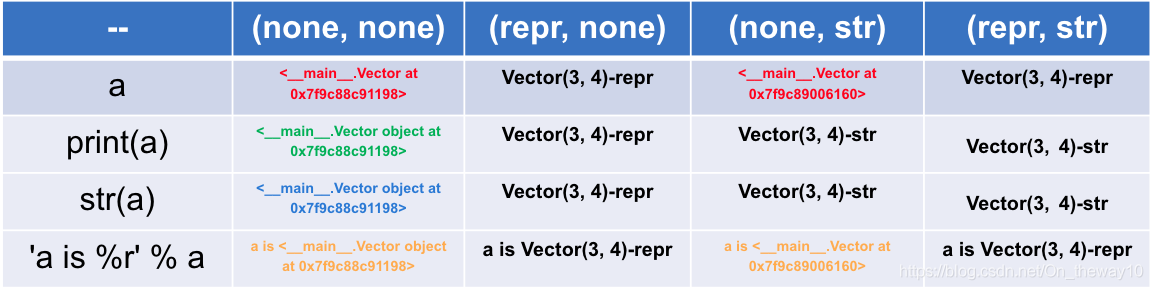# Python: ___ () vs ___ str__()

``````class Vector(object):

def __init__(self, x=0, y=0):
self.x = x
self.y = y

def __repr__(self):
return 'Vector(%r, %r)-repr' % (self.x, self.y)

def __abs__(self):
return 'Vector(%r, %r)-str' % (self.x, self.y)``````

ResultComment

The method of

• /repr__() is provided by the object class, and all Python classes are subclasses of the object class, so all Python objects have the method of ___. So if you need to concatenate any object with a string, you can call the method with ___ first to turn the object into a string and then concatenate the strings together.
• repr__() is special because it is a “self-describing” method, usually with the following scenario: when the developer prints the object directly, the system prints out the “self-describing” message with the object, telling the outside world the state the object has . __repr__() the method provided by the
• object always returns the “ class name +object at + memory address ” with the object implementation class. This return value does not really implement the ‘self-describing’ function, so if the user needs to custom class to do it, he/she must rewrite the method with repr__().
• is generally more readable with succinct __str__(), and the return result of each __repr__() is more accurate and more appropriate for the developer. </ li>
• for developers, in the implementation class, suggested rewriting __repr__ () function </ span> </ strong>!